Published: 10 October 2014

# Identification of the parameters of visco-elastic model of the hydraulic vibration absorber

Jan Warczek1
Jakub Młyńczak2
Rafal Burdzik3
Łukasz Konieczny4
1, 2, 3, 4Faculty of Transport, Silesian University of Technology, Krasińskiego Street 8, 40-019 Katowice, Poland
Corresponding Author:
Jan Warczek
Views 39

#### Abstract

The paper presents the results of visco-elastic dampers. Their aim was to determine the parameters of dynamic models. A key issue in modeling is to determine the acceptable degree of simplification. According to the authors diagnostic models must take into account characteristics such as nonlinearity and asymmetry damping characteristics. Also, it is important to take into account the apparent elasticity of the hydraulic damper. Sample parameters of dynamic models of automotive shock absorbers are presented in graphical form.

## 1. Introduction

The construction of diagnostic databases requires obtaining diagnostic information during the experiments on real objects or simulation studies. Studies of real objects are often time-consuming and very costly. The alternative, which are simulation studies, allows acquisition of diagnostic knowledge with the help of a computer and appropriate software. The task of diagnosing technical object often necessitates troubleshooting based on the early symptoms of the condition. In practice, the use of vibro-acoustic method involves the analysis of low-energy signals disorders. In this case, the necessary condition is to use a specific model. Model which best reflects how reality. Diagnosis according to the model opens up huge possibilities. Assess the current state of technical and analysis of the future development of damage allows the use of effective countermeasures. The great advantage of diagnostic simulation is also possible to optimize the process control operation [1-3].

The fundamental role it plays shock is associated with a reduction of unsprung masses relative movements and unsprung. The essence of the problem is that, despite the relative ease of construction of the shock absorber element of the vehicle is complex, non-linear dynamic properties. The aim of the study was to determine the model parameters of nonlinear viscous damper on the example of the automobile shock absorber.

## 2. Modeling of automotive vibration dampers

The suspension dynamics simulations vehicles commonly used procedure is to model the strut largely simplification. The greatest possible simplification of the model in the form of damping constant damping coefficient. Depending on the parent to conduct simulation studies, this approach is often justified, for example in simple models with two degrees of freedom used to test algorithms for vibration control.

Characteristics of real damper is non-linear and very often asymmetric with respect to the origin [2, 4, 5]. Non-linear damping of the relative velocity of the damper piston models using features (e.g. polynomial of $n$-th degree) is determined separately for the movement of reflection and deflection.

In modeling the nonlinear damping characteristics of the shock absorber uses the theory of spline functions. The non-linear damper model is defined by a set of basis points by which the characteristic curve is carried calculated using spline functions. Such models can be found in commercial programs for the analysis of dynamic phenomena occurring in vehicles.

In any previously reported example of modeling the damping characteristics assumed uniqueness of the instantaneous value of the force generated by the shock to the instantaneous speed of the relative motion of the piston and the housing of the damper. Assumption implies that the shock absorber car is part of a viscous damping force depends on the resistance to motion in all conditions only on the speed of extortion. This is a major limitation of the adequacy of the models discussed range of dynamic damper for a range of low-frequency excitations, the value of which does not exceed 0.5 [Hz]. For such excitations no trends apparent stiffness of the damper and the effect is negligible impact force amplitude jump on the shape of the damping characteristics.

For the analysis of the dynamics of the damper for higher frequency vibrations influence of those parameters is significant. The phenomenon of seeming stiffness of the damper test that measured the stand illustrated by graphs. The velocity obtained for a fixed length of the working stroke (Fig. 1).

Fig. 1The loop diagrams obtained for the force sinusoidal and various frequency extortion – visible effect of seeming stiffness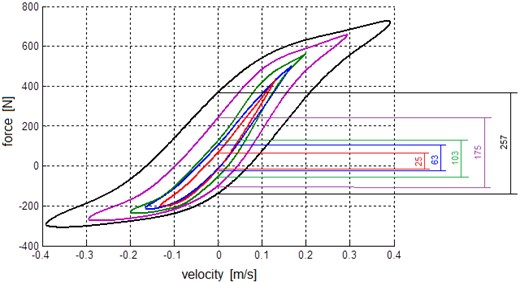The higher the force, the greater the value of seeming stiffness, as can be seen in the graphs in the form of velocity in their enlarged width near zero speed override. Thus, the force generated by the shock absorber is the sum of two components: seeming stiffness and damping [6, 7].

Another aspect of examining the accuracy of computational models this phenomenon impact shock stroke length to force the shape of the damping characteristics. In the case of a sinusoidal force maximum speed reciprocating motion of the piston damper always get the time for which the offset value is equal to zero, assuming that the origin of the coordinate system is positioned in the middle of the length of the working stroke. The maximum value of the speed force can be achieved in two ways – by increasing the length of the working stroke fixed frequency or force at a constant stroke length by changing the frequency of extortion. Designated by such methods damping characteristics demonstrate compliance only when using the low-frequency excitations. In real traffic conditions, vehicle vibration from a wide range of frequencies, and the speed of movement of the piston shock absorber of a few m/s are both long and short strokes working. For this reason, it is appropriate considering the damping characteristics of the shock absorber as a function of two-arguments.

Property shortly discussed hydraulic shock absorber raises a question regarding the required level of detail of the model dynamic damper, which will be the basis for the development of diagnostic measures. Pre in drawing up the model, the following assumptions:

– Taking into account seeming stiffness of the damper,

– Define the damping characteristics as a function of two-arguments.

## 3. Research of shock absorbers

Automotive shock absorbers test was carried out according to the scheme shown in Fig. 2. Used in the research kinematic sinusoidal force whose parameters such as duration and amplitude were chosen so that their combination gave the same values of the maximum velocity of movement extortion.

Fig. 2The scheme of measurement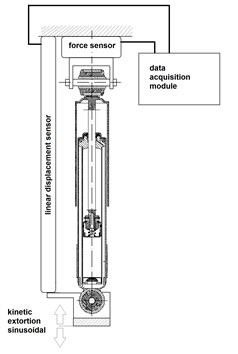Recording of results were made for each test frequency to give a series of files, which contain the forces generated by the shock absorber, the corresponding values of traverse of the piston damper and the sampling frequency. In order to determine the damping characteristics of the shock absorber to the charts work read maximum values of forces in tension and compression at times, for which the speed of the reciprocating motion of the kinematic force was maximal. In this way, for the working stroke of the shock absorber gives the series damping force values obtained for subsequent frequency domains. For each test pitch frequency domains were selected in such a way that each row in the table each time to obtain the same value of the maximum speed of movement.

Graphical representation of the shock absorber damping characteristics obtained for different strokes force is shown in Fig. 3 clearly shows the dependence of the damping characteristics of total stroke length extortion.

Fig. 3The set shock absorber damping characteristics obtained for a selected length of the working strokes of the damper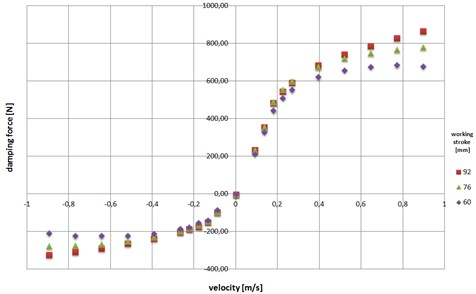The described procedure was achieved record parametric absorber damping properties set within a certain range of variation extortion. The strong dependence of the damping characteristics of the working stroke length determines the need to take account of this phenomenon in computational models of automotive shock absorbers. The relationship describing this phenomenon in general is as follows:

1
${F}_{D}=f\left(v,{w}_{s}\right),$

where: $v$ – the current velocity of extortion, ${w}_{s}$ – the total length of the stroke force.

The study also helped determine the relationship describing the apparent stiffness of the damper. For this purpose, an experiment was planned work involving the appointment of graphs obtained for a given frequency domains with different pitches working. For each test a combination of stroke length and frequency domains of work were read chart of the forces that were generated by the shock absorber in times of zero traffic speed extortion. Sample results are presented in Fig. 4.

Fig. 4The seeming stiffness of the damper characteristics obtained for different frequencies of extortion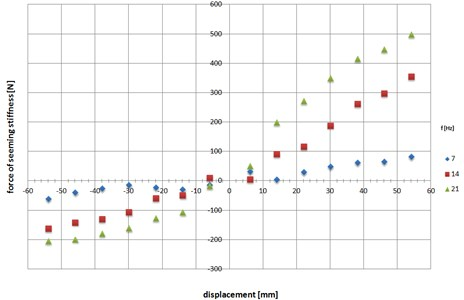Characteristics of seeming stiffness of the damper force is a function of frequency and displacement force. So we can force seeming stiffness of the damper represented as:

2
${F}_{ss}=f\left(d,f\right),$

where: $d$ – kinetically forced displacement, $f$ – frequency of extortion.

The effect of frequency domains damper way of working is very significant. Twice a change in the frequency of working strokes absorber results in almost 50 % increase in strength resulting from the elastic properties of the shock absorber. It should be noted that the ratio of the stiffness of the damper takes almost zero values for frequencies less than 0.5 Hz.

## 4. The visco-elastic model of the viscous vibration damper

Consideration of dependencies described in Eqs. (1) and (2) allows to introduce the viscous vibration damper model as a pair of graphs surface (Figs. 5 and 6).

Fig. 5The surface damping force values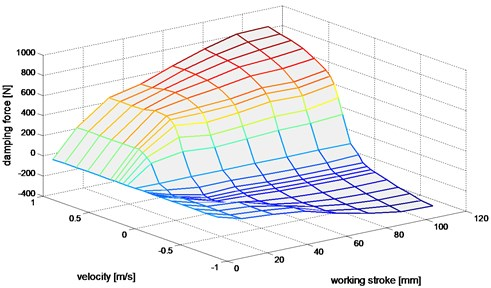Instantaneous damping forces and apparent modulus may be based on such a model set by interpolation. So the appointment of the approximate values of the function at points other than nodes and estimation error of the approximate values. To do this, find a function $F\left(x\right)$, called the interpolation function, which in the nodes of interpolation has the same values as the function $y=f\left(x\right)$.

Interpolation is in a sense opposite to the tabulation task functions. With the analytical form of the function build an array of values, while interpolation based on an array of functions we define its analytical form. According to the authors the best way to determine the values of the damping forces and apparent resilience is a two-argument function interpolation using the theory of spline functions.

Fig. 6The distribution of seeming stiffness values of forces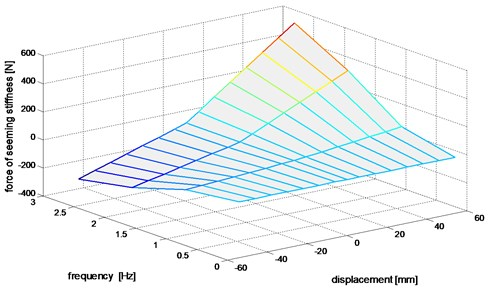## 5. Conclusions

The obtained results of the model of the shock absorber are not the whole issue, which is very extensive. The model to some extent closer signaled solution of the problem, which is the correct choice of the degree of simplification. In diagnostic applications, simulation an essential element of the process of building the knowledge base is to use the most detailed description of reality. This allows you to define a measure of the technical condition sensitive to minor amendments.